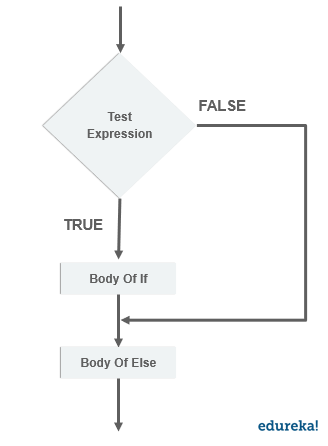# If Else In Python With Examples : Everything You Need To KnowPython programming language is extremely easy to learn. The lesser the lines of codes and reduced is the complexity. It provides readability and improved efficiency. Concepts like control statements and conditional statements help in clarity when a user wants to test multiple expressions. In this article we will discuss the concept of if and else in python. Following are the topics discussed in this article.

## What Are Python Conditions?

Conditions in python are logical conditions which we use in if and else statements. Following are some logical conditions in python.

1. Equal to – x == y
2. Not equal to – x != y
3. Less than – x < y
4. Less than or equal to – x <= y
5. Greater than – x > y
6. Greater than or equal to – x >= y

These are the conditions we often use while declaring a test expression for if and else statements in python.

## What Is ‘if’ And ‘else’ In Python?

An if statement is used to test an expression and execute certain statements accordingly. A program can have many if statements present in a program. The subsequent or the penultimate statement that follows is an else statement, which executes a statement in case all the test expressions in the program are false. To understand the control flow of an if and else statement, take a look at the flow chart of how the execution takes place.

## Flow Control And SyntaxIf you look carefully, you can figure out that the execution starts with the test expression, when it is true the execution will enter the body of if and execute all the statements. But when the expression is false, the execution will move to the body of else and execute the statements in the else block.

Just like an if statement, we can add an else if or elif statement in python to test out more expressions in order to optimize the code and increase the efficiency of our code. Lets take a look at the syntax to understand how we can declare a if else statement block in python.

Syntax:

```
if (test expression) :
# statement to be executed
elif (test expression 2):
# statements of elif block
else:
# final statement
```

To understand how we can use if else in python lets take a look at a program to find out if a number is even or odd.

```x = 10
if x % 2 == 0:
print('even number')
elif x % 2 == 1:
print('odd number')
else:
print('enter a number')
```
`Output : even number`

To check the number, we are using a logical condition in the test expression, which will check the remainder and if the remainder is 0 it will print the statement in the if block. Otherwise it will go to the next expression which is the elif statement. Since 10 divided by 2 leaves the remainder as 0, the output is ‘even number’.

Lets take another example of finding out a prime number using if else.

```x = 9
for i in range(2,x):
if i % 2 == 0:
print('not a prime number')
else:
print('prime number')
```

In this program, we are also making use of a for loop. If else can be used in various loops as well.

## Shorthand ‘if’ And ‘else’

If you have only one statement to execute you can put it in a single line.

```if a > b: print("greater ")
```

Short hand else

```print("greater") if a > b else print("smaller")
```

You can use multiple else statements as well.

```print("greater") if a > b else print("equal")  if a ==b else print("smaller")
```

Using logical operators in if else

```a = 10
b = 20
if a > b and a > 10 :
print("greater")
elif a < b or a < 10:
print("smaller")
else:
print("not a valid number")
```

## Use Case – Nested if else

```
x = 10
if x != 0:
if x % 2 == 0:
print('even number')
else:
print('odd number')
elif x == 0:
print('equal to zero')
elif x > 0:
for i in range(2,x):
if i % 2 == 0:
print('not a prime number')
else:
print('prime number')
else:
print('less than zero')
```

In this program we have used multiple test expression inside if statements. This is how we can use nested if else in python.

This article has covered all the concepts related to if else statements in python. With the use of conditional and control statements in python the code becomes efficient and optimized. Python programming language provides an easy syntax which results in improved readability and saves the effort in writing complex codes. With all the development in fields like artificial intelligence and machine learning python is becoming more desired by both- the companies and developers as well. To master all the skills related to python programming enroll to edureka’s python certification program and kickstart your learning.

Have any questions? mention them in the comments, our team will get back to you as soon as possible.

Upcoming Batches For Python Certification Training Course
Course NameDate
Python Certification Training Course

Class Starts on 28th May,2022

28th May

SAT&SUN (Weekend Batch)
View Details
Python Certification Training Course

Class Starts on 18th June,2022

18th June

SAT&SUN (Weekend Batch)
View DetailsREGISTER FOR FREE WEBINAR
Send OTPThank you for registering Join Edureka Meetup community for 100+ Free Webinars each month

### Subscribe to our Newsletter, and get personalized recommendations.#### If Else In Python With Examples : Everything You Need To Know

edureka.co